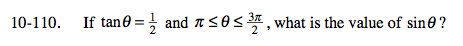### Home > A2C > Chapter Ch10 > Lesson 10.2.4 > Problem10-110

10-110.$\text{Remember that tan\theta=\frac{opp}{adj}. }$

Use the Pythagorean theorem to find the hypotenuse.

(hyp)2 = (2)2 + (1)2

$\text{hyp=\sqrt{5}}$

Be careful, θ is in the third quadrant, so sine is negative.

$\text{sin\theta=\frac{opp}{hyp}}$